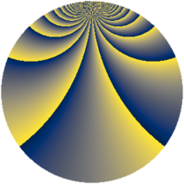# Properties

 Label 1014.2.rLevel $1014$ Weight $2$ Character orbit 1014.r Rep. character $\chi_{1014}(5,\cdot)$ Character field $\Q(\zeta_{52})$ Dimension $1488$ Sturm bound $364$

# Related objects

## Defining parameters

 Level: $$N$$ $$=$$ $$1014 = 2 \cdot 3 \cdot 13^{2}$$ Weight: $$k$$ $$=$$ $$2$$ Character orbit: $$[\chi]$$ $$=$$ 1014.r (of order $$52$$ and degree $$24$$) Character conductor: $$\operatorname{cond}(\chi)$$ $$=$$ $$507$$ Character field: $$\Q(\zeta_{52})$$ Sturm bound: $$364$$

## Dimensions

The following table gives the dimensions of various subspaces of $$M_{2}(1014, [\chi])$$.

Total New Old
Modular forms 4464 1488 2976
Cusp forms 4272 1488 2784
Eisenstein series 192 0 192

## Trace form

 $$1488q + 12q^{7} + O(q^{10})$$ $$1488q + 12q^{7} + 124q^{16} + 12q^{19} + 24q^{27} - 12q^{28} - 104q^{30} - 12q^{31} - 36q^{33} - 12q^{37} - 104q^{39} - 24q^{42} + 224q^{45} - 12q^{52} + 36q^{54} - 88q^{55} + 36q^{57} + 16q^{61} - 224q^{63} - 16q^{66} + 220q^{67} + 208q^{70} + 12q^{73} - 260q^{75} + 12q^{76} + 36q^{78} - 80q^{79} - 16q^{81} + 72q^{85} - 32q^{87} + 12q^{91} + 224q^{93} + 64q^{94} + 60q^{97} - 36q^{99} + O(q^{100})$$

## Decomposition of $$S_{2}^{\mathrm{new}}(1014, [\chi])$$ into newform subspaces

The newforms in this space have not yet been added to the LMFDB.

## Decomposition of $$S_{2}^{\mathrm{old}}(1014, [\chi])$$ into lower level spaces

$$S_{2}^{\mathrm{old}}(1014, [\chi]) \cong$$ $$S_{2}^{\mathrm{new}}(507, [\chi])$$$$^{\oplus 2}$$